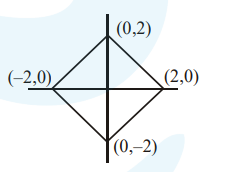# The region represented byQuestion:

The region represented by $|x-y| \leq 2$ and $|x+y| \leq 2$ is bounded by a :

1. square of side length $2 \sqrt{2}$ units

2. rhombus of side length 2 units

3. square of area $16 \mathrm{sq}$, units

4. rhombus of area $8 \sqrt{2}$ sq. units

Correct Option: 1

Solution:

$|x-y| \leq 2$ and $|x+y| \leq 2$Square whose side is $2 \sqrt{2}$